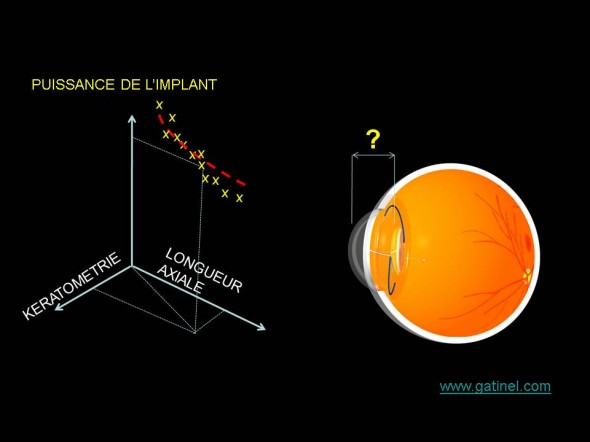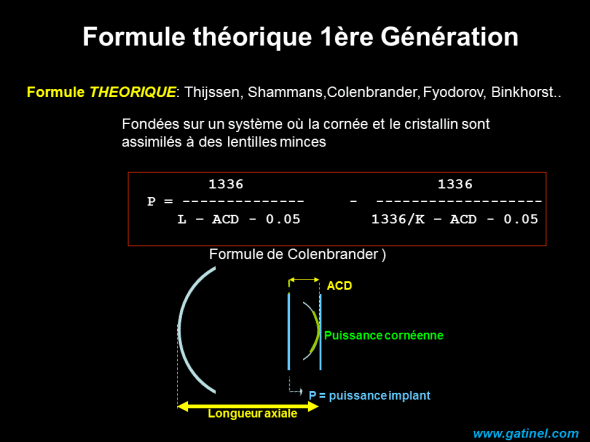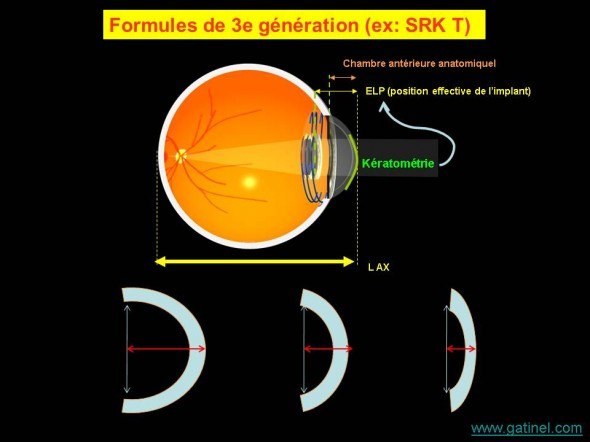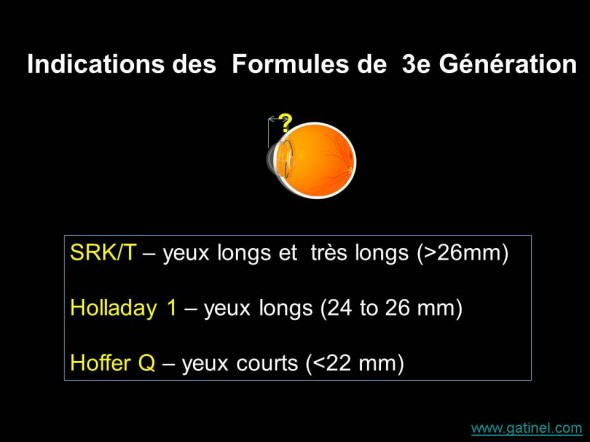# Implant power calculation formulas

Formulas that allow the calculation of the power of the implant prior require the collection of data specific to the operated eye. Biometrics allows the collection of the parameters required for the calculation of the power of the implant position to induce the refraction desired after the cataract surgery (replacement of the lens clouded by an artificial lens implant).

Obtaining this power depends on a calculation formula. There are several forms of biometric calculation, not only for historical reasons, but also because all the biometric calculation formulas are based on certain assumptions (for example, the prediction of actual position of the implant(, but also the index of refraction of the cornea and other eye circles, etc.). These assumptions are particularly based in certain types of eyes, but may be source of error in others.

Thus, some forms are rather recommended depending on the axial length (short, 22 mm, vs long > 24 mm eyes eyes) or the existence of history of corneal refractive surgery.

Two families of formulas are schematically distinguished: regression formulas, and theoretical (or "exact") formulas. In some classifications, regression formulas are classified as "second generation" formulas. In reality, there is always a part of "regression" in the theoretical formulas, because it is not possible to predict with certainty the exact position of the implant in the eye (its distance with the cornea) before the surgery.

## Regression formulas

The forms of so-called regression have been established as retrospective from data such as the measurement of the corneal power (keratometry), axial length, the placed implant, and finally obtained refraction power. Calculating regression aims to establish an equation that expresses the power of the implant from these so-called "predictors".Regression formulas are calculated based on the link between keratometry, axial length and power of the implant put in normalsighted patients after cataract surgery. They correspond to the equation of the curve which marries the best point cloud whose details match the keratometry, axial length in this example and the power of the placed implant. The dispersion of the values is related in large part to changes in the position of the implant in the eye.

An analogy with this method would be for a tailor to establish a formula to predict better the good suit size to its customers from the measurement of their size (in cm) and weight (in kg). With a database referencing hundreds of clients (and a good statistical software), it would be possible to this merchant to establish such a formula.

The first formula established at the end of the 1960s by Fyodorov wasn't a regression formula, but a formula theoretical first generation, based on a mathematical model of the eye where a path of light Ray was applied. The problems encountered with the first results, which were not only related to the formula but to technical measures, have not made this universal approach. The SRK formula was developed in the early 1980s as a regression formula by Sanders, Retzlaff, and Kraft:

P = A − 2.5 L − 0.9 K

This formula can be interpreted as the development series of Taylor (first degree) from a theoretical formula of first generation, where the power of the implant varies linearly based on variations of L (axial length) and K (keratometry).  With this development, we get a constant (A) whose value can be modulated to adjust better to the clinical data.  The value of the constant A is close to 118 for posterior Chamber implants. This constant is adjusted to limit the effect of variations in the actual position of the implant at best. L is the axial length (in mm) and K the keratometry average (diopter). The constant A is therefore related more or less directly to the anatomic depth of the anterior chamber (and its variations). At one time pioneer in artificial lens implant, the latter was fixed against the iris. Anatomical postoperative anterior chamber depth was very close to the position of the implant.

This SRK formula had the advantage of being simple, at a time the electronic and computer calculation was still inaccessible for clinicians.

Example of implant power calculation:

A = 116.8, L = 23mm and K = 43D, P = 116.8 57.5 − − 38.7 = 20.6D. This power is the power that is calculated for the community intra ocular (implant located "in the eye", index of refraction close to 1.33). In the air (index of refraction = 1), this power would be larger.

SRK is now obsolete, as it has proved to be relatively imprecise 'atypical' eye (the Taylor type approximations are accurate only around a small area of value, ex: 'means' eyes).  The relationship between these parameters is not linear, especially the influence of the axial length. It is interesting to understand the basic principles of the calculation provided by this formula (regression), because such an approach is still used to estimate the value of the actual position of the implant: given that it is not measurable in preoperative, and that only a predictive approach to type "statistics" can be used.

The theoretical formulas that are today used for biometric calculation are said to be "theoretical" because they are based on the application of formulas of vergence on a theoretical eye model. We then apply virtually a path of light rays (near the optical axis) through the various optical surfaces (which of the implant). In this calculation, it is necessary to assign a position to the implant: it's in the prediction of this position is the differences between the theoretical formulas 'modern '.

## Theoretical (or accurate) formulas of calculation of implant

The first theoretical formulas to calculate the power of the implant from a simplified eye model were baptized in honor of their Developer (this tradition continues). In Retrospect there are several generation of theoretical formulas, because a simple calculation using the formula of vergence underlines an important point: the position of the implant in the eye cannot be known with certainty before the surgery (the implant can be positioned a few hundred microns forwards or backwards in the capsular bag).

Can be grouped classify formulas based on their generation, this classification is subject to variations according to the authors.

The simplest classification distinguishes the theoretical formulas tell first-generation, empirical formulas - second generation (see above), and the forms of theoretical modern - so-called third generation.

### Theoretical formulas of first generation

The formula of Fyodorov was a pioneer, and followed by the formulas of Colebrander (1973), Hoffer (1974) and Binkhorst (1975) (C D Binkhorst was a Dutch ophthalmologist who developed a model of implant clipped to the iris). Other authors have proposed a close theoretical formula: formula for Thijssen, Van der Heijde formula... Despite a sometimes different analytical expression in the numerator and denominator, these formulas are almost (or even completely) identical (Thijseen formula takes into account the thickness of the implant, the formula of Colebrander to the position of principal of the cornea plans, etc.)The theoretical formulas are based on the use of a simplified look and vergences forms model. The value used for the actual position of the implant (C) is necessarily arbitrary. It understandable that the subsequent formulas have had as main engine to increase the accuracy of the prediction of the actual position of the implant. The Colebrander formula is slightly modified with respect to the theoretical formula obtained by calculation of vergence: the actual position of the implant is increased from 0.05 mm, the distance corresponding to the position of the plan main purpose of the cornea - see power of the cornea paraxial optics).

The theoretical formulas are based on the use of a simplified look and vergences forms model. The value used for the actual position of the implant (ACD for "Anterior Chamber Depth") is necessarily arbitrary. The formula of Binkhorst II used a predicted proportion to the axial length value of ACD:

Postop ACD = CDA pre-op x (L/23.45)

It understandable that the subsequent formulas have had as main engine to increase the accuracy of the prediction of the actual position of the implant. The Colebrander formula is slightly modified with respect to the theoretical formula obtained by calculation of vergence: the actual position of the implant is increased from 0.05 mm, the distance corresponding to the position of the plan main purpose of the cornea - see power of the cornea paraxial optics).

Modern theoretical formulas are based on these original formulas: These exact formulations differ in the way in which they predict the actual position of the implant post surgery (that is to say the position of the implant in the eye after the surgery). Formulas of first generation experienced mixed success because the axial length measurement techniques were not as accurate as today ' hui: we used at the time a contact method where a probe was transmitting Ultrasonics in contact with the cornea: the speed of propagation of ultrasound could fluctuate depending on the degree of cataract, and the examiner, in involuntary pressing on the cornea during the measurement could artificially reduce the value of the axial length.

The introduction of interferometric measurement in 1999 (IOL Master, Zeiss), as well as the theoretical formulas of second generation (ie the third generation formulas!), where the value of the actual position of the implant is a function of various parameters (and allows a better prediction of the power of the implant) have generalized the use of theoretical formulas.

Hoffer was in 1982 one of the first to try to correlate the actual position of the implant to the axial length: the longer eyes generally have a deeper than the shorter eyes anterior Chamber. This relationship between axial length and depth of the anterior chamber sets the formulas of second generation.

The relationship used by Hoffer to connect the position of the implant with the axial length was:

ELP = 2.93 x AL - 2.92

### Theoretical forms of so-called third generation

They are characterized by the use of the keratometry to perfect the prediction of the actual position of the implant.3rd generation formulas are based on the use of special algorithms to improve the prediction of the actual position of the implant from the value of the keratometry; a more arched cornea suggests an arrow (so a room depth) increased.

Holladay suggested using the corneal curvature as a preacher of the final position of the implant, emphasizing the difference between the value of depth of anterior Chamber ' iris 'aACD"for Anterior Chamber Depth corneal Summit) and the distance between corneal vertex and implant. The difference (distance iris implant) was named "Sucker Factor" (SF). The calculation of the value of SF could then involve the object of regression by the constant A of the implant. Depth of anterior Chamber is predicted from a regression on the axial length and the keratometry.

#### Formula SRK T

It is inspired by this approach that Retzlaff (the ' R' of the trio of authors at the origin of the acronym SRK) proposed the SRK T (T for theoretical) formula, but retaining the concept of constant A (probably for not to annoy many users of the SRK regression formula). This A constant was converted into effective position of the implant on a linear formula.

ELP corneal arrow + 0.62467 = x - 68.747 - A 3.336

The corneal arrow is a parameter that allows you to connect the remote iris/cornea corneal curvature. From this estimate arises a problem of refractive surgery surgery patients: the corneal curvature is changed surgically, and is no longer a good preacher of the depth of the anterior Chamber.

#### Hoffer formula Q

Similarly, Hoffer proposed in 1992 Hoffer Q formula, using a quadratic formula to predict a depth of anterior chamber theoretical custom from the keratometry; Once obtained, it was then used as the basis for a function of the keratometry and axial length adjustment.

3rd generation formulas are used in routine cataract surgery. They particularly Excel in some eye conformations (short eyes, long eyes, etc.). The following figure summarizes preferential directions of these formulas, depending on the axial length of the eyeball concerned.The axial length generally determines the choice of the 3rd generation used for biometric calculation formula

The latest 3rd generation formulas give less (or even no) emphasis on keratometry for the calculation of the position predicted the implant (ELP). This makes them particularly useful in situations where the keratometry was altered surgically, either for the eyes having benefited in the past from surgery (refractive)LASIK(, PKR, radiaire keratotomii, etc.)

#### Formula of Olsen

It is based on the use of various parameters of the anterior segment, as the depth of the anterior chamber (anatomical) preoperative to establish an algorithm using this value to improve the predictability of the calculation of the actual position of the implant. In this formula, the influence of the keratometry on the actual position of the implant is zero.

#### Haigis formula

In 1999, Haigis suggested using 3 constants to predict the actual position of the implant, based on the anatomical features of the eye and the used implant. As the formula of Olsen, the keratometry is not used to predict the actual position of the implant. On the other hand, the type of implant, the depth of the anterior chamber (preoperative) and axial length are included in the calculation of the actual position of the implant (ELP).

Some formulas are not published verbatim (Holladay II) or are the subject of more theoretical than practical applications (ray tracing). Some authors consider these formulas as of 4th or 5th generation.

Hollday has developed a formula where various anatomical parameters of the anterior segment of the eye as the diameter white to white (diameter of the cornea), as well as the age of the patient, the sex, the preoperative refraction are used to predict a value called ESF for Etimated Scaling Factor. This value is then multiplied by the effective position of the implant predicted by the manufacturer. This formula, proposed in 1996, has never been published. It is available on internet through a paid subscription.

Barrett Universal II Formula

The Barrett's formula is based on the use of the keratometry, the axial length, the depth of the anterior chamber. The white to white diameter (sclérocornéen blade diameter) and the thickness of the Crystalline lens are optional data. Barrett also developed a formula for calculating the cylinder's power (astigmatism) of the o-implants, and a formula designed to more accurately estimate the overall corneal power (Barrett true K).

Artificial Intelligence/deep learning.

there cataract surgery is the most performed procedure in number, all surgical procedures combined. There are hundreds of thousands or even millions of potentially usable data, to improve existing formulas, i.e. to compensate for the uncertainty regarding the position of the implant (effective lens position) in the eye at the end of the intervention. The use of "intelligent" algorithms, or rather made efficient by the mass of data that feed them, is a promising track in ocular biometrics.

The FOrmule Hill-RBF  Represents a first approach in the field. It is however designed from the data of a particular biometer (Haag-Streit Lenstar) and two types of monofocal intraocular lenses. However, it is possible to extrapolate the results of the calculation to other types of lenses, and to use the data of competing biometers to perform the calculations.

We have also worked on the development of a formula based on the integration of data by an artificial intelligence algorithm in order to further improve the accuracy of the biometrics calculation of the eyes operated by cataract surgery , and presented the preliminary results of this work (awarded with an award) at the ASCRS Congress in may 2019 (San Diego, USA). We made a new biometric formula: PEARL DGS). This formula is designed to improve the accuracy of biometrics, especially for previously operated refractive surgery eyes.

#### Ray tracing

The use of a software capable to perform the simulation of the path of rays through a model eye, including corneal surface can be given by of topographic measurements before the operation (anterior, posterior, thickness of the cornea). The ray tracing allows a calculation of throwing rays outside of the terms paraxiales (Gauss optics). As a result, the ray tracing takes into account the asphericity of the corneal surface. On the other hand, like other optical formulas, the actual position of the implant cannot be known in advance.  This type of calculation is not frequently used, because it requires the use of specific software (Oculix) and the superiority of this approach on the formulas of third generation has not been demonstrated.

### 3 Responses to "Implant power calculation Formulas"

1.SAMMY RCHMOND says:

THANK YOU ENORMOUSLY FOR THESE INFORMAIONS ON THE DIFFERENT CALCULATION OF THE IMPLANTS INTR EYE.

THIS HELPS ME A LOT AS A SPECIALIST ENGINEER OF OPHTHALMOLOGIC QUIPENMENTS IN COTE D'IVOIRE AND IN THE SUB-REGIONS

2.Manon says:

Hello
For patients with laser refractive surgery, the Haigis formula would be the most suitable because it does not take into account the keratometry?
For an eye with a standart axial length I use the formula SRK-T, or you say that it is rather used for a very long eye, which it formula should we use then?

3.Dr. Damien Gatinel says: